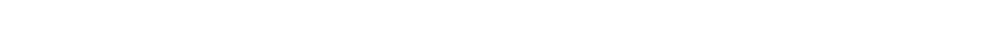#si',\$content,\$matches); //var_dump(\$matches); foreach (\$matches as \$key => \$p) { if(preg_match('/color:\s*#333399;/si',\$p)){ \$conArr[]['tit'] = preg_replace('/<[^<]+?>/si', '', \$p); }else{ if(count(\$conArr) != 0){ \$conArr[count(\$conArr)-1]['step'] .= \$p; } } } //var_dump(\$conArr); return \$conArr; } \$cats = array_merge(subcategory(\$catid)); foreach(\$cats as \$k => \$cat){ \$cats[\$k]['sub'] = array_merge(subcategory(\$cat['catid'])); } foreach (\$cats as \$k => \$cat) { foreach (\$cat['sub'] as \$i => \$subcat) { if(preg_match('/\d+\$/',\$subcat['abbr'],\$match)){ \$cats[\$k]['sub'][\$i]['steps'] = getContent(\$match); } } } //var_dump(\$cats); ?>

# 深圳之窗

## 办理地点

• 深圳摇号网上申请地址：《深圳小汽车增量调控管理信息系统

• 深圳汽车竞价参加地址：《深圳小汽车增量指标竞价平台

• 关于国税信息的有关问题咨询：关于国税的问题，请选择以下任何一种方式咨询：（1）电话咨询：请拨打深圳市国税局咨询热线12366. （2）网站咨询：请登陆深圳市国税局网址查询（http://www.szgs.gov.cn/）（3）现场咨询：前往国税办税大厅咨询，可通过国税局网址查询办税大厅地址。

• 关于地税信息的有关问题：《深圳小汽车增量指标竞价平台

## 常见问题